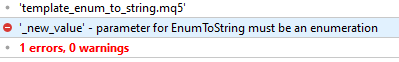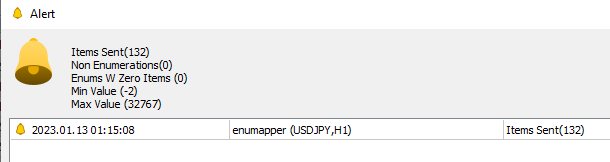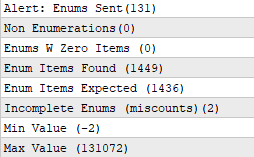# EnumToString and turning values to string in a template class

If you have something similar and each type of data is handled (in the example only double and enum because its an example) and

you want to turn the value to text automatically , i.e. new value , instant format to text ,why is it not allowed to use the function for enumerations (EnumToString) when you create a double type instance of the class .

Can't it assume i'm handling it by types ?```template <typename X>
class anything{
X value;
string as_text;
public:
anything(void){as_text=NULL;}
void set(X _new_value){
value=_new_value;
if(typename(_new_value)=="double"){as_text=DoubleToString(value,2);}
//if its an enum
else if(StringFind(typename(_new_value),"enum",0)!=-1){
as_text=EnumToString(_new_value);
}
}
string text(){return(as_text);}
};
int OnInit()
{
//---
anything <double>f;
double v=1.23;
f.set(v);
Print(f.text());
//---
return(INIT_SUCCEEDED);
}```

Thanks

Edit , the opposite is allowed :

```  anything <ENUM_APPLIED_PRICE>f;
ENUM_APPLIED_PRICE v=PRICE_TYPICAL;
f.set(v);
Print(f.text());```

Do you know this to print variable name and its value:

```#define TOSTR(A)         #A+"=" + (string)(A)
int i = 7;
Print( TOSTR(i));```

Someone has already posted it here or in an article.

Carl Schreiber #:

Do you know this to print variable name and its value:

Someone has already posted it here or in an article.

Interesting trick . Thanks . (you can grab the variable name for better error reporting with this !  🤯 🖤 )

The goal is to have an object of any type which holds its text value automatically.

The current workaround is this :

```template <typename X>
class anything{
X value;
string as_text;
public:
anything(void){as_text=NULL;}
void set(X _new_value){
value=_new_value;
if(typename(_new_value)=="double"){as_text=DoubleToString(value,2);}
}
string text(){return(as_text);}
template <typename F>
string text_ets(F type){
return(EnumToString((F)value));
}
};
int OnInit()
{
//---

anything <double>f1;
double v1=1.23;
f1.set(v1);
Print(f1.text());

anything <ENUM_APPLIED_PRICE>f2;
ENUM_APPLIED_PRICE v2=PRICE_TYPICAL;
f2.set(v2);
Print(f2.text_ets((ENUM_APPLIED_PRICE)0));
//---
return(INIT_SUCCEEDED);
}```

the problem is it negates the reason for its existence if the end user (who will also be a coder) has to constantly pass the enum type

also tried to have a "sampler" which would peacefully reside inside the object without hitting template mismatch errors

but it went no-where so , if there was a trick to turn the typen (string) to a type that would be it  😋

```class sampler{
public:
string typen;
};

template <typename X>
class sampler_specific : public sampler{
public:
X ptr;
sampler_specific(void){typen=typename(ptr);}
};

sampler *ptrs[];

int OnInit()
{
//new specific sampler

sampler_specific<int> *s=new sampler_specific<int>();
ArrayResize(ptrs,1,0);
ptrs=GetPointer(s);
Print(ptrs.typen);

return(INIT_SUCCEEDED);
}```

There is another alternative , to grab the enum strings prior to a setup and throw them in alongside their values (of the enumeration) and do matching .

i've resorted to this enumeration value grabber and the hypothesis is that the default enums range from SHORT_MIN to SHORT_MAX in values .

If this is wrong i'd appreciate a notice.

```template <typename X>
int full_enumeration_grab(X enumeration,
short &result_codes[],
string &results[],
int first_value=SHORT_MIN,
int last_value=SHORT_MAX){
int enum_values=0;
ArrayResize(result_codes,1000,0);
if(is_enumeration(enumeration)){
//set the first value
X en=(X)first_value;
string name="";
for(int i=SHORT_MIN;i<=SHORT_MAX;i++)
{
en=(X)((short)i);
name=EnumToString(en);
//if valid
if(StringFind(name,"::",0)==-1){
enum_values++;
result_codes[enum_values-1]=i;
}
}
//grab the values
if(enum_values>0){
ArrayResize(result_codes,enum_values,0);
ArrayResize(results,enum_values,0);
for(int i=0;i<enum_values;i++){
results[i]=EnumToString((X)(result_codes[i]));
}
}else{
ArrayFree(result_codes);
}
}
return(enum_values);
}```

amrali #:
Use UINT_MAX+1000

for the first value or the last value?

amrali #:
0 to UINT_MAX+1000

i dont think i can loop that much

If this is wrong i'd appreciate a notice.

Anyway!

i'm sorry, it is bed time now.

0 to USHORT_MAX+1000

Edit: I deleted my non-sense replies.

amrali #:

Anyway!

i'm sorry, it is bed time now.

0 to USHORT_MAX+1000

Edit: I deleted my non-sense replies.

goodnight

I decided to do a test .132 default enumerations (all of them i think)

Out of which all were enumerations (NonEnumerations = 0)

Out of which all had at least one item detected (Enums W Zero Items =0)

Min Value is reported to be -2 and max value 32767 so

There may be enums missing on the upside for each enumeration , but the -2 to int_max test will take too long probably.

I'm attaching the code for scrutiny of any mistakes that i'm not aware of.

(the only probable mistake is there are more enums above 32767)

```int OnInit()
{
//---
EventSetMillisecondTimer(333);
items_sent=0;
non_enumerations=0;
enums_with_zero_items=0;
max_value=INT_MIN;
min_value=INT_MAX;
//---
return(INIT_SUCCEEDED);
}

void OnTimer(void)
{
EventKillTimer();
int f=FileOpen("enumap.txt",FILE_WRITE|FILE_TXT);
if(f!=INVALID_HANDLE){
write_enum_to_file(f,(ENUM_ACCOUNT_INFO_DOUBLE)0);
write_enum_to_file(f,(ENUM_ACCOUNT_INFO_INTEGER)0);
write_enum_to_file(f,(ENUM_ACCOUNT_INFO_STRING)0);
write_enum_to_file(f,(ENUM_ACCOUNT_MARGIN_MODE)0);
write_enum_to_file(f,(ENUM_ACCOUNT_STOPOUT_MODE)0);
write_enum_to_file(f,(ENUM_ACTIVATION_FUNCTION)0);
write_enum_to_file(f,(ENUM_ALIGN_MODE)0);
write_enum_to_file(f,(ENUM_ANCHOR_POINT)0);
write_enum_to_file(f,(ENUM_APPLIED_VOLUME)0);
write_enum_to_file(f,(ENUM_ARROW_ANCHOR)0);
write_enum_to_file(f,(ENUM_BASE_CORNER)0);
write_enum_to_file(f,(ENUM_BOOK_TYPE)0);
write_enum_to_file(f,(ENUM_BORDER_TYPE)0);
write_enum_to_file(f,(ENUM_CALENDAR_EVENT_FREQUENCY)0);
write_enum_to_file(f,(ENUM_CALENDAR_EVENT_IMPACT)0);
write_enum_to_file(f,(ENUM_CALENDAR_EVENT_IMPORTANCE)0);
write_enum_to_file(f,(ENUM_CALENDAR_EVENT_MULTIPLIER)0);
write_enum_to_file(f,(ENUM_CALENDAR_EVENT_SECTOR)0);
write_enum_to_file(f,(ENUM_CALENDAR_EVENT_TIMEMODE)0);
write_enum_to_file(f,(ENUM_CALENDAR_EVENT_TYPE)0);
write_enum_to_file(f,(ENUM_CALENDAR_EVENT_UNIT)0);
write_enum_to_file(f,(ENUM_CHART_EVENT)0);
write_enum_to_file(f,(ENUM_CHART_MODE)0);
write_enum_to_file(f,(ENUM_CHART_POSITION)0);
write_enum_to_file(f,(ENUM_CHART_PROPERTY_DOUBLE)0);
write_enum_to_file(f,(ENUM_CHART_PROPERTY_INTEGER)0);
write_enum_to_file(f,(ENUM_CHART_PROPERTY_STRING)0);
write_enum_to_file(f,(ENUM_CHART_VOLUME_MODE)0);
write_enum_to_file(f,(ENUM_CL_DEVICE_TYPE)0);
write_enum_to_file(f,(ENUM_COLOR_FORMAT)0);
write_enum_to_file(f,(ENUM_COPY_RATES)0);
write_enum_to_file(f,(ENUM_COPY_TICKS)0);
write_enum_to_file(f,(ENUM_CRYPT_METHOD)0);
write_enum_to_file(f,(ENUM_CUSTOMIND_PROPERTY_DOUBLE)0);
write_enum_to_file(f,(ENUM_CUSTOMIND_PROPERTY_INTEGER)0);
write_enum_to_file(f,(ENUM_CUSTOMIND_PROPERTY_STRING)0);
write_enum_to_file(f,(ENUM_DATABASE_EXPORT_FLAGS)0);
write_enum_to_file(f,(ENUM_DATABASE_FIELD_TYPE)0);
write_enum_to_file(f,(ENUM_DATABASE_IMPORT_FLAGS)0);
write_enum_to_file(f,(ENUM_DATABASE_OPEN_FLAGS)0);
write_enum_to_file(f,(ENUM_DATABASE_PRINT_FLAGS)0);
write_enum_to_file(f,(ENUM_DATATYPE)0);
write_enum_to_file(f,(ENUM_DAY_OF_WEEK)0);
write_enum_to_file(f,(ENUM_DEAL_ENTRY)0);
write_enum_to_file(f,(ENUM_DEAL_PROPERTY_DOUBLE)0);
write_enum_to_file(f,(ENUM_DEAL_PROPERTY_INTEGER)0);
write_enum_to_file(f,(ENUM_DEAL_PROPERTY_STRING)0);
write_enum_to_file(f,(ENUM_DEAL_REASON)0);
write_enum_to_file(f,(ENUM_DEAL_TYPE)0);
write_enum_to_file(f,(ENUM_DRAW_TYPE)0);
write_enum_to_file(f,(ENUM_DX_BUFFER_TYPE)0);
write_enum_to_file(f,(ENUM_DX_FORMAT)0);
write_enum_to_file(f,(ENUM_DX_HANDLE_TYPE)0);
write_enum_to_file(f,(ENUM_DX_PRIMITIVE_TOPOLOGY)0);
write_enum_to_file(f,(ENUM_ELLIOT_WAVE_DEGREE)0);
write_enum_to_file(f,(ENUM_FILESELECT_FLAGS)0);
write_enum_to_file(f,(ENUM_FILE_POSITION)0);
write_enum_to_file(f,(ENUM_FILE_PROPERTY_INTEGER)0);
write_enum_to_file(f,(ENUM_FP_CLASS)0);
write_enum_to_file(f,(ENUM_GANN_DIRECTION)0);
write_enum_to_file(f,(ENUM_GEMM)0);
write_enum_to_file(f,(ENUM_INDEXBUFFER_TYPE)0);
write_enum_to_file(f,(ENUM_INDICATOR)0);
write_enum_to_file(f,(ENUM_INIT_RETCODE)0);
write_enum_to_file(f,(ENUM_LINE_STYLE)0);
write_enum_to_file(f,(ENUM_LOSS_FUNCTION)0);
write_enum_to_file(f,(ENUM_MATRIX_NORM)0);
write_enum_to_file(f,(ENUM_MA_METHOD)0);
write_enum_to_file(f,(ENUM_MQL_INFO_INTEGER)0);
write_enum_to_file(f,(ENUM_MQL_INFO_STRING)0);
write_enum_to_file(f,(ENUM_OBJECT)0);
write_enum_to_file(f,(ENUM_OBJECT_PROPERTY_DOUBLE)0);
write_enum_to_file(f,(ENUM_OBJECT_PROPERTY_INTEGER)0);
write_enum_to_file(f,(ENUM_OBJECT_PROPERTY_STRING)0);
write_enum_to_file(f,(ENUM_OPENCL_EXECUTION_STATUS)0);
write_enum_to_file(f,(ENUM_OPENCL_HANDLE_TYPE)0);
write_enum_to_file(f,(ENUM_OPENCL_PROPERTY_INTEGER)0);
write_enum_to_file(f,(ENUM_OPENCL_PROPERTY_STRING)0);
write_enum_to_file(f,(ENUM_ORDER_PROPERTY_DOUBLE)0);
write_enum_to_file(f,(ENUM_ORDER_PROPERTY_INTEGER)0);
write_enum_to_file(f,(ENUM_ORDER_PROPERTY_STRING)0);
write_enum_to_file(f,(ENUM_ORDER_REASON)0);
write_enum_to_file(f,(ENUM_ORDER_STATE)0);
write_enum_to_file(f,(ENUM_ORDER_TYPE)0);
write_enum_to_file(f,(ENUM_ORDER_TYPE_FILLING)0);
write_enum_to_file(f,(ENUM_ORDER_TYPE_TIME)0);
write_enum_to_file(f,(ENUM_PLOT_PROPERTY_DOUBLE)0);
write_enum_to_file(f,(ENUM_PLOT_PROPERTY_INTEGER)0);
write_enum_to_file(f,(ENUM_PLOT_PROPERTY_STRING)0);
write_enum_to_file(f,(ENUM_POINTER_TYPE)0);
write_enum_to_file(f,(ENUM_POSITION_PROPERTY_DOUBLE)0);
write_enum_to_file(f,(ENUM_POSITION_PROPERTY_INTEGER)0);
write_enum_to_file(f,(ENUM_POSITION_PROPERTY_STRING)0);
write_enum_to_file(f,(ENUM_POSITION_REASON)0);
write_enum_to_file(f,(ENUM_POSITION_TYPE)0);
write_enum_to_file(f,(ENUM_PROGRAM_TYPE)0);
write_enum_to_file(f,(ENUM_REGRESSION_METRIC)0);
write_enum_to_file(f,(ENUM_SERIESMODE)0);
write_enum_to_file(f,(ENUM_SERIES_INFO_INTEGER)0);
write_enum_to_file(f,(ENUM_SIGNAL_BASE_DOUBLE)0);
write_enum_to_file(f,(ENUM_SIGNAL_BASE_INTEGER)0);
write_enum_to_file(f,(ENUM_SIGNAL_BASE_STRING)0);
write_enum_to_file(f,(ENUM_SIGNAL_INFO_DOUBLE)0);
write_enum_to_file(f,(ENUM_SIGNAL_INFO_INTEGER)0);
write_enum_to_file(f,(ENUM_SIGNAL_INFO_STRING)0);
write_enum_to_file(f,(ENUM_SORT_MODE)0);
write_enum_to_file(f,(ENUM_STATISTICS)0);
write_enum_to_file(f,(ENUM_STO_PRICE)0);
write_enum_to_file(f,(ENUM_SYMBOL_CALC_MODE)0);
write_enum_to_file(f,(ENUM_SYMBOL_CHART_MODE)0);
write_enum_to_file(f,(ENUM_SYMBOL_INDUSTRY)0);
write_enum_to_file(f,(ENUM_SYMBOL_INFO_DOUBLE)0);
write_enum_to_file(f,(ENUM_SYMBOL_INFO_INTEGER)0);
write_enum_to_file(f,(ENUM_SYMBOL_INFO_STRING)0);
write_enum_to_file(f,(ENUM_SYMBOL_OPTION_MODE)0);
write_enum_to_file(f,(ENUM_SYMBOL_OPTION_RIGHT)0);
write_enum_to_file(f,(ENUM_SYMBOL_ORDER_GTC_MODE)0);
write_enum_to_file(f,(ENUM_SYMBOL_SECTOR)0);
write_enum_to_file(f,(ENUM_SYMBOL_SWAP_MODE)0);
write_enum_to_file(f,(ENUM_TERMINAL_INFO_DOUBLE)0);
write_enum_to_file(f,(ENUM_TERMINAL_INFO_INTEGER)0);
write_enum_to_file(f,(ENUM_TERMINAL_INFO_STRING)0);
write_enum_to_file(f,(ENUM_TIMEFRAMES)0);
write_enum_to_file(f,(ENUM_VECTOR_CONVOLVE)0);
write_enum_to_file(f,(ENUM_VECTOR_NORM)0);
FileClose(f);
string msg="Items Sent("+IntegerToString(items_sent)+")\n";
msg+="Non Enumerations("+IntegerToString(non_enumerations)+")\n";
msg+="Enums W Zero Items ("+IntegerToString(enums_with_zero_items)+")\n";
msg+="Min Value ("+IntegerToString(min_value)+")\n";
msg+="Max Value ("+IntegerToString(max_value)+")\n";
}
}

int items_sent=0;
int non_enumerations=0;
int enums_with_zero_items=0;
int max_value=INT_MIN,min_value=INT_MAX;
template <typename X>
void write_enum_to_file(int file_handle,X enumeration){
items_sent++;
string result_enums[];
int  result_codes[];
int total=full_enumeration_grab(enumeration,result_codes,result_enums,SHORT_MIN,SHORT_MAX);
FileWriteString(file_handle,typename(enumeration)+" ("+IntegerToString(total)+")\n");
if(total>0){
for(int i=0;i<total;i++){
FileWriteString(file_handle,result_enums[i]+" ["+IntegerToString(result_codes[i])+"]\n");
}}else{
enums_with_zero_items++;
}
FileWriteString(file_handle,"--------------------------\n");
}

template <typename X>
int full_enumeration_grab(X enumeration,
int &result_codes[],
string &results[],
int first_value=INT_MIN,
int last_value=INT_MAX){
int enum_values=0;
ArrayResize(result_codes,1000,0);
if(is_enumeration(enumeration)){
//set the first value
X en=(X)first_value;
string name="";
for(int i=first_value;i<=last_value;i++)
{
en=(X)(i);
name=EnumToString(en);
//if valid
if(StringFind(name,"::",0)==-1){
enum_values++;
result_codes[enum_values-1]=i;
if(i>max_value){max_value=i;}
if(i<min_value){min_value=i;}
}
}
//grab the values
if(enum_values>0){
ArrayResize(result_codes,enum_values,0);
ArrayResize(results,enum_values,0);
for(int i=0;i<enum_values;i++){
results[i]=EnumToString((X)(result_codes[i]));
}
}else{
ArrayFree(result_codes);
}
}else{non_enumerations++;}
return(enum_values);
}
template <typename X>
bool is_enumeration(X &object){
/*
default and custom enum typenames have enum and then the name of the enum
*/
if(StringFind(typename(object),"enum",0)!=-1){return(true);}
return(false);
}```

Update , counted (with mistakes :p) the enum items , and the found enums are above the estimate so , it is possible to then get all the values encountered in an array 1500 possible values

which will be used to detect the enumeration choices of any enumeration .

Min value -2 max value 131072 , i miscounted at least 13 enum items but i dont think it goes higher (the mapper reports 2 miscounts , if you want to advance it you can detect which ones)

I think that is it .

I 'm attaching the update file if someone wants to checkedit , i stopped sending enum ENUM_GEMM as i could not find any docs or info about it
Files:
enumapper.mq5  15 kb